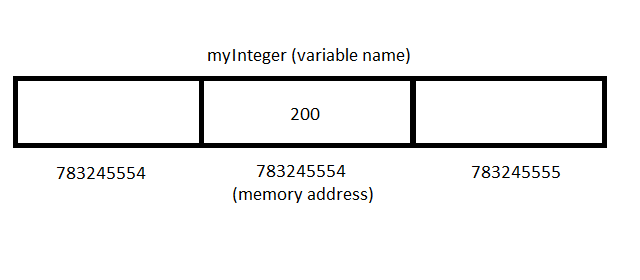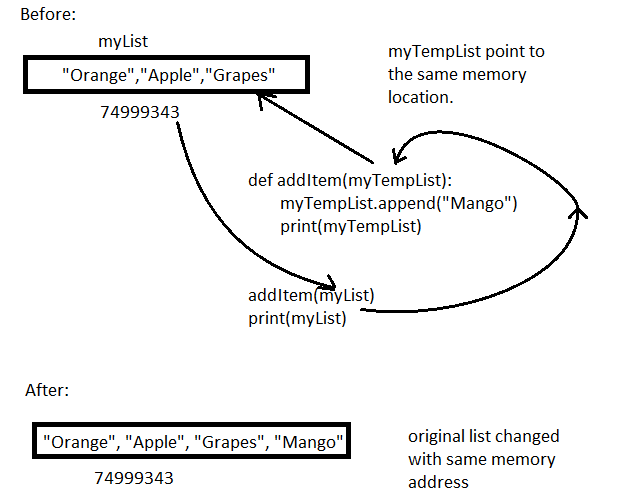# Python Passing-By-Reference vs. Passing-By-Value

In earlier articles, you have learned about python functions and their types. In this article, you will learn about passing-by-reference vs. passing-by-value in python. This concept is already well known in many programming languages.

We will understand the difference between passing-by-reference vs. passing-by-value with respect to python programming language.

Before we begin, you must understand how variables are stored in python.

## How Variables Are Stored in Memory ?

A `variable `is simply a container in python, an `identifier `that points to some data value. Each data item has a data types associated with it. A data type can be mutable or immutable, about which we will discuss later.

Suppose we declared a variable – `myInteger `and assigned it value of `200`. See the figure below where each box is a memory location.Python Variable Memory Representation

A python variable points to a memory location and the value of the variable is stored in that location. Each memory location is identified by its own memory address.

## Passing-By-Reference

By default, python pass the variable to a function as a reference. In this technique, the memory location of the variable is sent to the function.

If the function is able to change the original value of the variable using the reference. We call it passing-by-reference.

Consider following example.

``````# A new list with memory location
myList = ["Orange", "Apple","Grapes"]

# Function to add an item to the list
def addItem(myTempList):
myTempList.append("Mango")
print(myTempList)
# Print the original list
addItem(myList)
print(myList)``````

The program add takes the argument `myList, `and `myTempList `in the function definition also points to `myList `data. Both of them points to same memory location.

Output of the program is given below.

``````['Orange', 'Apple', 'Grapes', 'Mango']
['Orange', 'Apple', 'Grapes', 'Mango']``````

The output shows that the original myList and the myTempList are same. This is the effect of passing by reference as it changes the original data.passing by reference changes the original variable data

Note: A data type must be mutable to be able to change original data.

## Passing By Value in Python

Python support passing by value which means only value of the variable is send to the function. Therefore, it is not going to affect the original variable.

The result of the computation is stored in a new memory location.

For example.

``````myInt = 45
print("myInt",id(myInt))
mySecondInt = myInt
print("mySecondInt",id(mySecondInt))
myInt = 10
print("myInt",id(myInt))
myInt += 23
print("myInt",id(myInt))``````

There are 3 variables in the above code – `myInt `and `mySecondInt.`

`mySecondInt `is holds the same value as `myInt`. In the next line, we changed the value of `myInt `to 10. The output of the program is given below.

``````myInt 140721280886544
mySecondInt 140721280886544
myInt 140721280885424
myInt 140721280886160``````

The program prints memory address of `myInt `and `mySecondInt `which is the same address.

The variable `myInt `is re-declared and it changed the memory address of the variable. It did not change the original value. Also, when `myInt `is incremented by 23, it did not change the value, but created a new `myInt `with a different memory location.

There are two takeaway from this:

• Declaration change the variable with new memory address.
• Primitive operation on variable changed its memory rather than changing the original variable.

The above is the nature of passing by value. The passing by value do not change the original value, but create a new one.Passing by Value does not change the original value and creates a new variable with new memory address

Consider the following program which uses passing by value.

``````myInt = 5
def addNumber(myNum):
myNum += 10
print(myNum)
print("Memory Address of myNum",id(myNum))

addNumber(myInt)
print(myInt)
print("Memory Address of myInt",id(myInt))``````

The output of the program is given below.

``````15
Memory Address of myNum 140721280885584
5
Memory Address of myInt 140721280885264
``````

Note that the value and memory address of the `myNum `and `myInt `is totally different. The original variable `myInt `was passed to the function, however, it was never changed.

This is passing by value which does not affect the original passed arguments.

### Summary

• Python by default pass by reference
• The pass by reference change the original value of variable passed as arguments. The variable must be mutable.
• Pass by value does not change the original variables passed to the function, and make a new variable. The data type must be immutable.